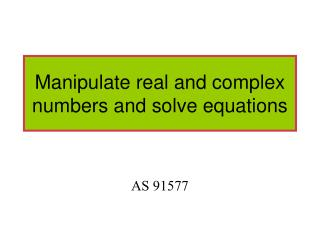Download PresentationManipulate real and complex numbers and solve equations

# Manipulate real and complex numbers and solve equations

Télécharger la présentation## Manipulate real and complex numbers and solve equations

- - - - - - - - - - - - - - - - - - - - - - - - - - - E N D - - - - - - - - - - - - - - - - - - - - - - - - - - -
##### Presentation Transcript

1. Worksheet 1

2. Quadratics General formula: General solution:

3. Example 1 Equation cannot be factorised.

4. Using quadratic formula We use the substitution A complex number

5. The equation has 2 complex solutions Imaginary Real

6. Equation has 2 complex solutions.

7. Example 2

8. Example 2

9. Example 2

10. Adding complex numbers Subtracting complex numbers

11. Example

12. Example

13. (x + yi)(u + vi) = (xu – yv) + (xv + yu)i. Multiplying Complex Numbers

14. Example

15. Example

16. Example 2

17. Conjugate If The conjugate of z is If The conjugate of z is

18. Dividing Complex Numbers

19. Example

20. Example

21. Example

22. Solving by matching terms Match real and imaginary Real Imaginary

23. Solving polynomials Quadratics: 2 solutions 2 real roots 2 complex roots

24. If coefficients are all real, imaginary roots are in conjugate pairs

25. If coefficients are all real, imaginary roots are in conjugate pairs

26. Cubic Cubics: 3 solutions 3 real roots 1 real and 2 complex roots

27. Quartic Quartic: 4 solutions 2 real and 2 imaginary roots 4 real roots 4 imaginary roots

28. Solving a cubic This cubic must have at least 1 real solutions Form the quadratic. Solve the quadratic for the other solutions x = 1, -1 - i, 1 + i

29. Finding other solutions when you are given one solution. Because coefficients are real, roots come in conjugate pairs so Form the quadratic i.e. Form the cubic:

30. Argand Diagram

31. Just mark the spot with a cross

32. z = i z = -1 z =1 z = -i

33. Reciprocal of z Conjugate

34. Rectangular to polar form Using Pythagoras Modulus is the length Argument is the angle Check the quadrant of the complex number

35. Modulus is the length

36. Example 1 Rectangular form Polar form

37. Example 2

38. Example 3

39. Converting from polar to rectangular

40. Multiplying numbers in polar form Example 1

41. Multiplying numbers in polar form Example 2 Take out multiples of